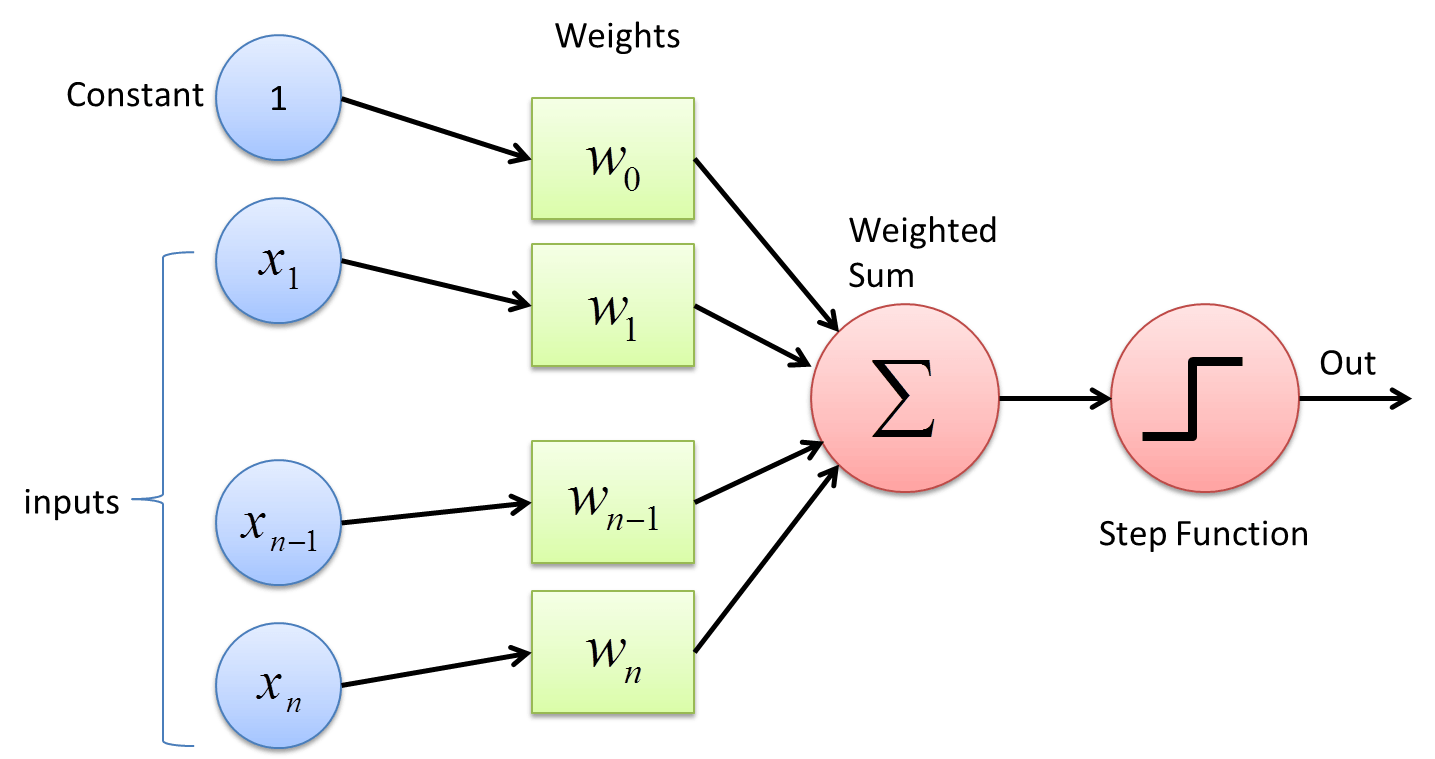# Implementing a Neuron in Analog Circuit

The fundamental unit of computation in a neural network is a perceptron or a logistic unit. Over past couple of months, I have been looking at analog implementation of perceptron.  Here is a simple architecture for it:(Image source: towardsdatascience.com)

The above architecture can be implemented in an analog circuit using OpAmps as shown below:Here, First OpAmp is implementing a summer. The output of it is given as:

Vs = – { (RF/R0) x 1 + (RF/R1) x X1 + (RF/R2) x X2 + (RF/R3) x X3 + (RF/R4) x X4 }

As one can see, weights w0 = (RF/R0), w1 = (RF/R1), w2 = (RF/ R2) …

V0 is a thresholding of Vs with a very low threshold by employing an inverting schmitt trigger.

By employing memristors in place of R0, R1, R2, R3 and R4. This can be made a trainable logistic unit. Connecting many such units we can make a neural network in analog. The advantage of such neurons are their speed and parallelism. They can operate even when inputs are available only partially.